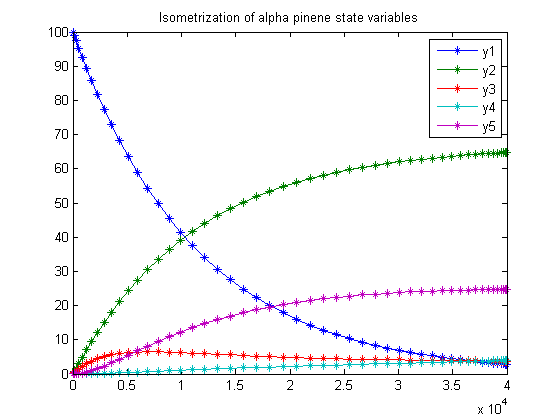53  Isometrization of alpha pinene

Benchmarking Optimization Software with COPS Elizabeth D. Dolan and Jorge J. More ARGONNE NATIONAL LABORATORY

53.1  Problem Formulation

Find theta over t in [0; 40000 ] to minimize

J =
 4 ∑ i=1

 8 ∑ j=1
(yj,i − ymeasj,i)2

subject to:

 dy1 dt
= −(theta1+theta2)*y1
 dy2 dt
= theta1*y1
 dy3 dt
= theta2*y1−(theta3+theta4)*y3+theta5*y5
 dy4 dt
= theta3*y3
 dy5 dt
= theta4*y3theta5*y5

 theta >= 0
 timemeas = [1230  3060  4920  7800  10680  15030  22620  36420]
 y1meas = [88.35  76.4  65.1  50.4  37.5  25.9  14.0  4.5]
 y2meas = [7.3  15.6  23.1  32.9  42.7  49.1  57.4  63.1]
 y3meas = [2.3  4.5  5.3  6.0  6.0  5.9  5.1  3.8]
 y4meas = [0.4  0.7  1.1  1.5  1.9  2.2  2.6  2.9]
 y5meas = [1.75  2.8  5.8  9.3  12.0  17.0  21.0  25.7]
 y0 = [100  0  0  0  0]

Reference: 

53.2  Problem setup

toms t theta1 theta2 theta3 theta4 theta5

53.3  Solve the problem, using a successively larger number collocation points

for n=[20 50]
p = tomPhase('p', t, 0, 40000, n);
setPhase(p);
tomStates y1 y2 y3 y4 y5

% Initial guess
if n == 20
x0 = {theta1 == 0; theta2 == 0
theta3 == 0; theta4 == 0
theta5 == 0; icollocate({
y1 == 100; y2 == 0
y3 == 0;   y4 == 0
y5 == 0})};
else
x0 = {theta1 == theta1opt; theta2 == theta2opt
theta3 == theta3opt; theta4 == theta4opt
theta5 == theta5opt; icollocate({
y1 == y1opt; y2 == y2opt
y3 == y3opt; y4 == y4opt
y5 == y5opt})};
end

% Box constraints
cbox = {0 <= theta1; 0 <= theta2; 0 <= theta3
0 <= theta4; 0 <= theta5};

% Boundary constraints
cbnd = initial({y1 == 100; y2 == 0
y3 == 0; y4 == 0; y5 == 0});

y1meas = [88.35; 76.4; 65.1; 50.4; 37.5; 25.9; 14.0; 4.5];
y2meas = [7.3; 15.6; 23.1; 32.9; 42.7; 49.1; 57.4; 63.1];
y3meas = [2.3; 4.5; 5.3; 6.0; 6.0; 5.9; 5.1; 3.8];
y4meas = [0.4; 0.7; 1.1; 1.5; 1.9; 2.2; 2.6; 2.9];
y5meas = [1.75; 2.8; 5.8; 9.3; 12.0; 17.0; 21.0; 25.7];
tmeas  = [1230; 3060; 4920; 7800; 10680; 15030; 22620; 36420];

y1err = sum((atPoints(tmeas,y1) - y1meas).^2);
y2err = sum((atPoints(tmeas,y2) - y2meas).^2);
y3err = sum((atPoints(tmeas,y3) - y3meas).^2);
y4err = sum((atPoints(tmeas,y4) - y4meas).^2);
y5err = sum((atPoints(tmeas,y5) - y5meas).^2);

% ODEs and path constraints
ceq = collocate({
dot(y1) == -(theta1+theta2)*y1
dot(y2) == theta1*y1
dot(y3) == theta2*y1-(theta3+theta4)*y3+theta5*y5
dot(y4) == theta3*y3
dot(y5) == theta4*y3-theta5*y5});

% Objective
objective = y1err+y2err+y3err+y4err+y5err;

53.4  Solve the problem

options = struct;
options.name = 'Isometrization of alpha pinene';
solution = ezsolve(objective, {cbox, cbnd, ceq}, x0, options);

% Optimal y and theta - starting guess in the next iteration
y1opt = subs(y1, solution);
y2opt = subs(y2, solution);
y3opt = subs(y3, solution);
y4opt = subs(y4, solution);
y5opt = subs(y5, solution);
theta1opt = subs(theta1, solution);
theta2opt = subs(theta2, solution);
theta3opt = subs(theta3, solution);
theta4opt = subs(theta4, solution);
theta5opt = subs(theta5, solution);
Problem type appears to be: qpcon
Starting numeric solver
===== * * * =================================================================== * * *
TOMLAB - Tomlab Optimization Inc. Development license  999001. Valid to 2011-02-05
=====================================================================================
Problem: ---  1: Isometrization of alpha pinene  f_k      19.872166933768312000
sum(|constr|)      0.000000000005482115
f(x_k) + sum(|constr|)     19.872166933773794000
f(x_0)   7569.999999999995500000

Solver: snopt.  EXIT=0.  INFORM=1.
SNOPT 7.2-5 NLP code
Optimality conditions satisfied

FuncEv    1 ConstrEv   74 ConJacEv   74 Iter   57 MinorIter  239
CPU time: 0.343750 sec. Elapsed time: 0.391000 sec.
Problem type appears to be: qpcon
Starting numeric solver
===== * * * =================================================================== * * *
TOMLAB - Tomlab Optimization Inc. Development license  999001. Valid to 2011-02-05
=====================================================================================
Problem: ---  1: Isometrization of alpha pinene  f_k      19.872166934168490000
sum(|constr|)      0.000000000010589892
f(x_k) + sum(|constr|)     19.872166934179081000
f(x_0) -38011.572833066202000000

Solver: snopt.  EXIT=0.  INFORM=1.
SNOPT 7.2-5 NLP code
Optimality conditions satisfied

FuncEv    1 ConstrEv   15 ConJacEv   15 Iter   11 MinorIter  263
CPU time: 0.328125 sec. Elapsed time: 0.359000 sec.
end

t  = subs(collocate(t),solution);
y1 = collocate(y1opt);
y2 = collocate(y2opt);
y3 = collocate(y3opt);
y4 = collocate(y4opt);
y5 = collocate(y5opt);

53.5  Plot result

figure(1)
plot(t,y1,'*-',t,y2,'*-',t,y3,'*-',t,y4,'*-',t,y5,'*-');
legend('y1','y2','y3','y4','y5');
title('Isometrization of alpha pinene state variables');